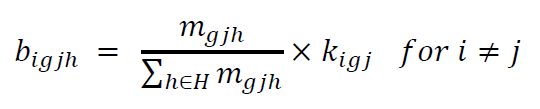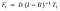# Computing Factor Content of Trade using the Proportionality Assumption with Python

After understanding factor content of trade, a natural protraction is calculating it. The following article presents a step-by-step implementation in python using the proportionality assumption.

The proportionality assumption is used as the required disaggregation of data is not available. In this case, the assumption is applied to trade flows where they follow a proportional ripple across the economy. That ripple can be expressed mathematically through :Where 𝑚 represents entries in the imported input-output matrix, 𝑘 represents the bilateral trade flows, and indices 𝑖, 𝑔 𝑗, ℎ represent the output country, output sector, input country, and input sector.

The imported input-output matrix (m) and the bilateral trade flows (k) can be obtained from GTAP9, a database gathered by the Global Trade Analysis Project at Purdue University. It consists of National input-output, trade, macroeconomic, and protection data tables assembled from the World Bank and IMF macroeconomic and Balance of Payments statistics, United Nations Commodity Trade Statistics (Comtrade) Database, and national statistical agencies.

That same database will also provide domestic input-output matrices and factor demand matrices required to compute the formulation of factor content of trade used by Reimer (2006) and Trefler and Zhu (2010):Where 𝐷 is the matrix of direct factor unit requirements, 𝐵 is the input-output matrix, (𝐼−𝐵)^−1 is the Leontief inverse, and 𝑇𝑖 is country 𝑖’s net trade, which is defined as the difference between exports and imports and represents direct and indirect factor inputs by 𝑋𝑖 — 𝑀𝑖.

For more details on the logic and intuition behind these formulas, please refer to Proportionality Assumption in Factor Content of Trade.

The first step consists in transforming data from GTAP9 into a format that allows for factor content of trade computations.

Firstly, domestic input-output matrices (VDFM in GTAP9) are transformed from a 3-dimensional array table with dimensions of output sector, input sector, and output/input country, into VDFMigjh, a collection of domestic input-output matrices for each country.

Secondly, bilateral trade flow vectors (VXMD in GTAP9) are transformed from a 3-dimensional array table with dimensions of output sector, output country, and input country, into VXMDigj, a collection of bilateral trade flow vectors for each country.

Thirdly, imported input-output vectors (VIFM in GTAP9) are transformed from a 3-dimensional array table with dimensions of output sector, input sector, and input country, into VIFMgjh, a 2-dimensional array table of import input-output vectors for each country.

Fourthly, factor demand matrices (VFM in GTAP9) are transformed from a 3-dimensional array table with dimensions of factor, sector, and country, into VFMfjh, a collection of factor demand matrices for each country.

Fifthly, indices for output sector (g), input sector (j), output country and output sector (𝑖𝑔), input country and input sector (𝑗ℎ), and factors (f) are extracted from datasets to iterate over in following steps.

The second step consists in outlining the bilateral trade and imported input-output constraints.

Bilateral trade constraints are built from the VXMDigj dataframe by separating constraints for each sector g and country j.

Input-output constraints are built from the VIFMgjh dataframe by separating constraints for each sector g and country j.

Once constraints are determined, we can impute values in the matrix B using the proportionality assumption.

The next steps consists in building the trade matrix based on the proportional ripple of imports across the economy.

Once all the data is imputed and in a usable format, we can compute factor content of trade as described by by Reimer (2006) and Trefler and Zhu (2010).

In short, most of the steps are required to get the data in a usable format and imputing imported input-output matrices proportionally. Afterwards, the computation of factor content of trade is rather straightforward.

Understanding the World through numbers | Senior Consultant — Omnia AI

## More from Peter Puszko

Understanding the World through numbers | Senior Consultant — Omnia AI